# Mole Concept - Concept of Limiting Reagent - Stoichiometric calculations

Stoichiometry deals with the calculation of the quantities of various reactants and products involved in a chemical reaction. The numerals used to balance a chemical equation are known as stoichiometric coefficients. These numerals are essential for solving problems based on chemical equation, hence called stoichiometric calculation.

In stoichiometric calculations, the mole relationship between different reactants and products are required as from them the mass-mass, mass-volume and volume-volume relationship between different reactants and products can be obtained.

Stoichiometric calculations can be carried out by two methods; Gravimetric Analysis and Volumetric Analysis. In the first method the amount of chemical species is determined by measurement of mass, whereas, in second method it is determined by measurement of volume.

Stoichiometric calculation can be carried in terms of two important concept:

(i) Mole Concept

(ii) Equivalent concept.

Mole Concept

A mole (symbol mol) is defined as the amount of substance that contains as many atoms, molecules, ions electrons or any other elementary entities as there are carbon atoms in exactly 12 gm of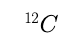. The number of atoms in 12 gm ofis called Avogadro’s number (N0).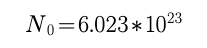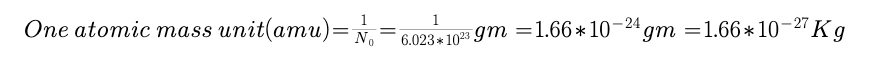The number of moles of a substance can be calculated by various means depending on data available, as follows.

Number of moles of molecules =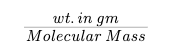Number of moles of atoms  =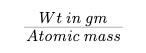Number of moles of gases =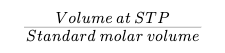Number of moles of particles e.g. atoms, molecules ions etc.  =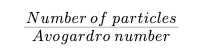Number of moles of solute = molarity *   volume of solution in litres =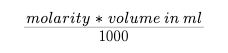for a compound AxBy, y moles of A = x moles of B

Mole fraction = fraction of the substance in the mixture expressed in terms of mol is called its mol fraction (X)

E.g. for a mixture of substance A & B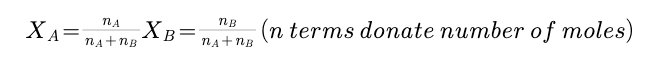Principle of Atom Conservation (POAC)

In chemical reaction atoms are conserved, so moles of atoms shall also be conserved. This is known as principle of atomic conservation. This principle is helpful in solving problems of nearly all stoichiometric calculations e.g.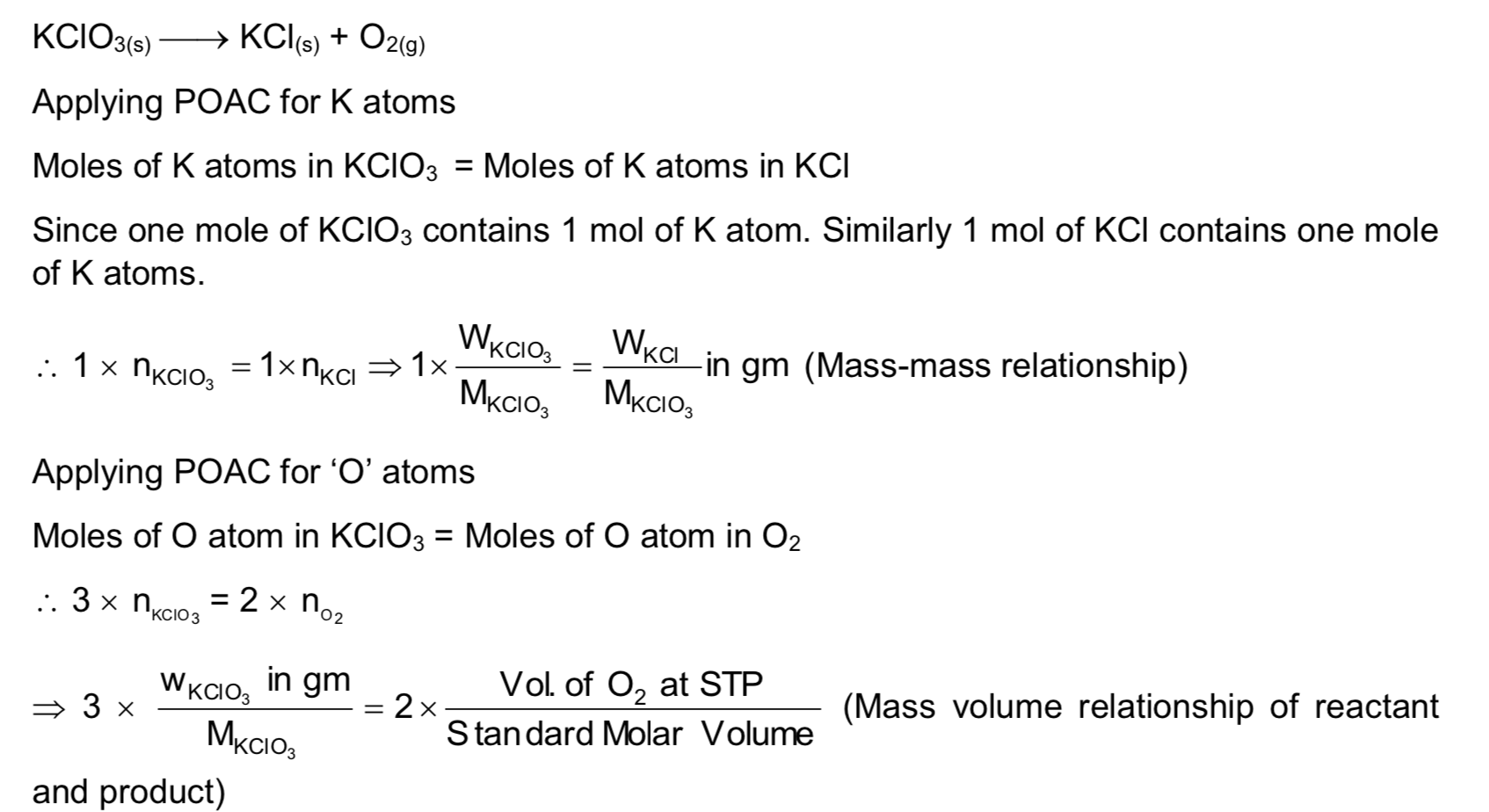In this way applying POAC we can break the chemical equation into a number arithmetic equations without balancing the chemical equation. Moreover number of reactions and their sequence from reactants to products are not required. It is important to note that POAC can be applied for the atoms which remain conserved in chemical reaction.

Concept of Limiting Reagent

In reactions involving more than one reactant, one has to identify first, of all the reactant, which is completely consumed (limiting reagent), one can identify the limiting reagent as follow: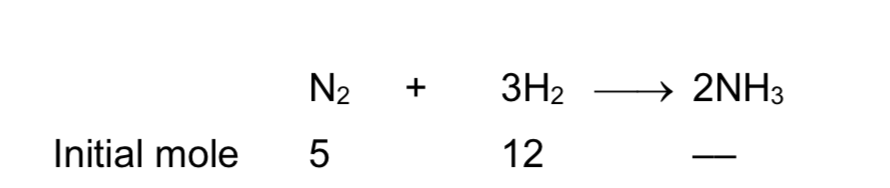If N2 is the limiting reactant moles of NH3 produced = 10. If H2 is the limiting reactant moles of NH3 produced = 32 12 = 8. The reactant producing the least number of mole of the product is the limiting reactant, hence H2 is the limiting reactant.

The limiting reactant can also be ascertained by knowing the initial number of equivalents or milli equivalents of each reactant. The reactant with least number of equivalents or milli equivalent is the limiting reactant. The equivalent methods to identify the limiting reactant used not require balancing of chemical equation.

Post By : Preeti Rai 12 Mar, 2020 3595 views Chemistry